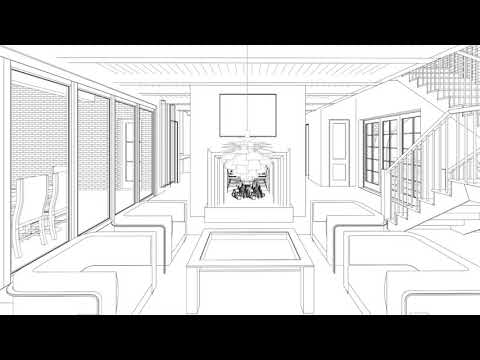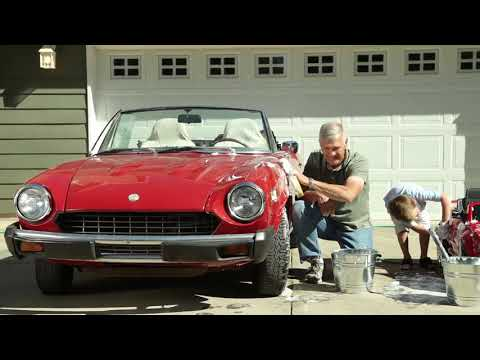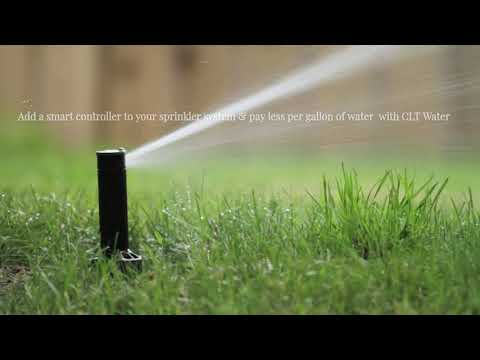```/* <![CDATA[ */
\$(function(){
var width = \$(window).innerWidth();
var setwidth = 640;
var ratio = 0.75;
var height = 480;
if(width < setwidth)
{
height = Math.floor(width * 0.75);
//console.log("device width "+width+", set width "+640+", ratio "+0.75+", new height "+ height);
var new_url = wpvl_paramReplace('width', link, width);
var new_url = wpvl_paramReplace('height', new_url, height);
\$("a#5f0cba2404211").attr('href', new_url);
//console.log(new_url);
}
});
});
/* ]]&gt; *//* <![CDATA[ */
\$(function(){
var width = \$(window).innerWidth();
var setwidth = 640;
var ratio = 0.75;
var height = 480;
if(width < setwidth)
{
height = Math.floor(width * 0.75);
//console.log("device width "+width+", set width "+640+", ratio "+0.75+", new height "+ height);
var new_url = wpvl_paramReplace('width', link, width);
var new_url = wpvl_paramReplace('height', new_url, height);
\$("a#5f0cba240424e").attr('href', new_url);
//console.log(new_url);
}
});
});
/* ]]&gt; *//* <![CDATA[ */
\$(function(){
var width = \$(window).innerWidth();
var setwidth = 640;
var ratio = 0.75;
var height = 480;
if(width < setwidth)
{
height = Math.floor(width * 0.75);
//console.log("device width "+width+", set width "+640+", ratio "+0.75+", new height "+ height);
var new_url = wpvl_paramReplace('width', link, width);
var new_url = wpvl_paramReplace('height', new_url, height);
\$("a#5f0cba240426e").attr('href', new_url);
//console.log(new_url);
}
});
});
/* ]]&gt; *//* <![CDATA[ */
\$(function(){
var width = \$(window).innerWidth();
var setwidth = 640;
var ratio = 0.75;
var height = 480;
if(width < setwidth)
{
height = Math.floor(width * 0.75);
//console.log("device width "+width+", set width "+640+", ratio "+0.75+", new height "+ height);
var new_url = wpvl_paramReplace('width', link, width);
var new_url = wpvl_paramReplace('height', new_url, height);
\$("a#5f0cba240428a").attr('href', new_url);
//console.log(new_url);
}
});
});
/* ]]&gt; *//* <![CDATA[ */
\$(function(){
var width = \$(window).innerWidth();
var setwidth = 640;
var ratio = 0.75;
var height = 480;
if(width < setwidth)
{
height = Math.floor(width * 0.75);
//console.log("device width "+width+", set width "+640+", ratio "+0.75+", new height "+ height);
var new_url = wpvl_paramReplace('width', link, width);
var new_url = wpvl_paramReplace('height', new_url, height);
\$("a#5f0cba24042a3").attr('href', new_url);
//console.log(new_url);
}
});
});
/* ]]&gt; */

```